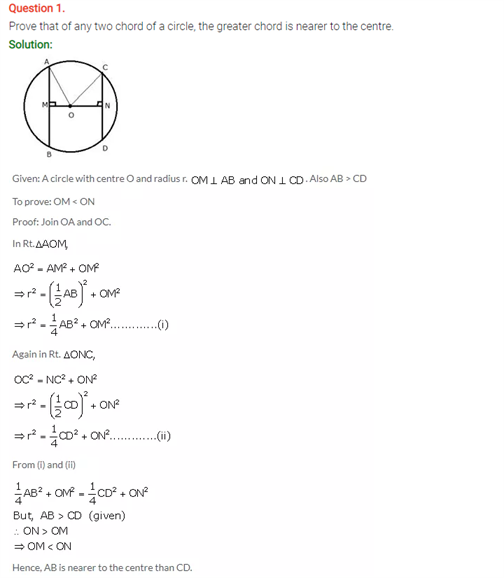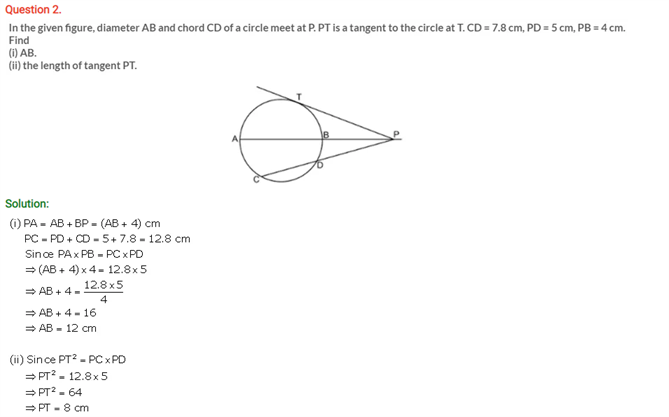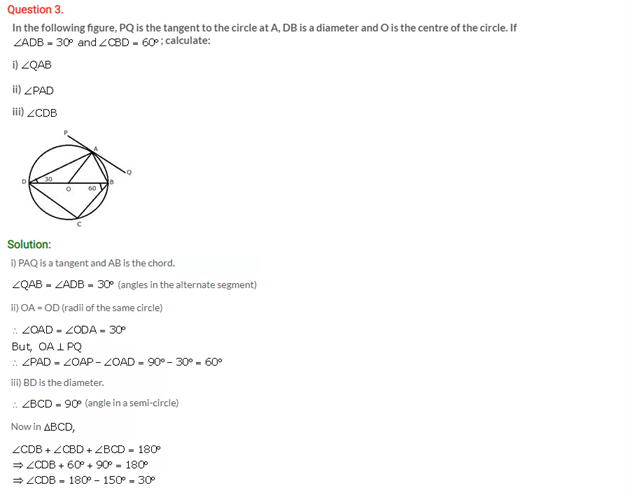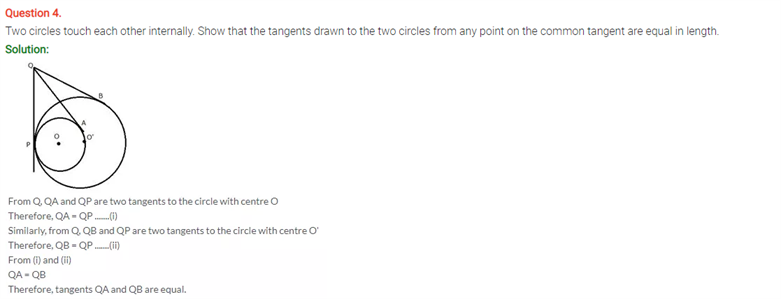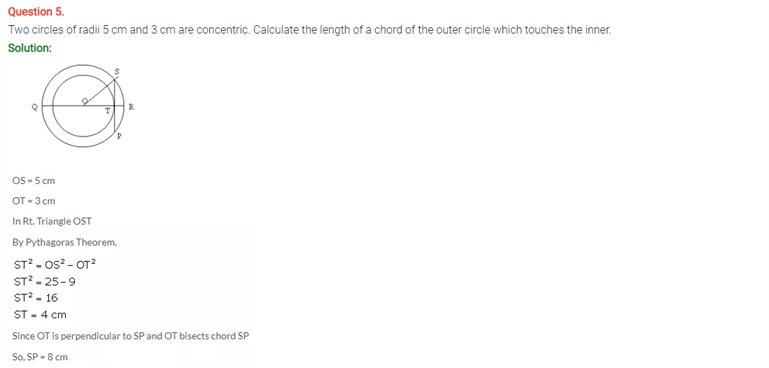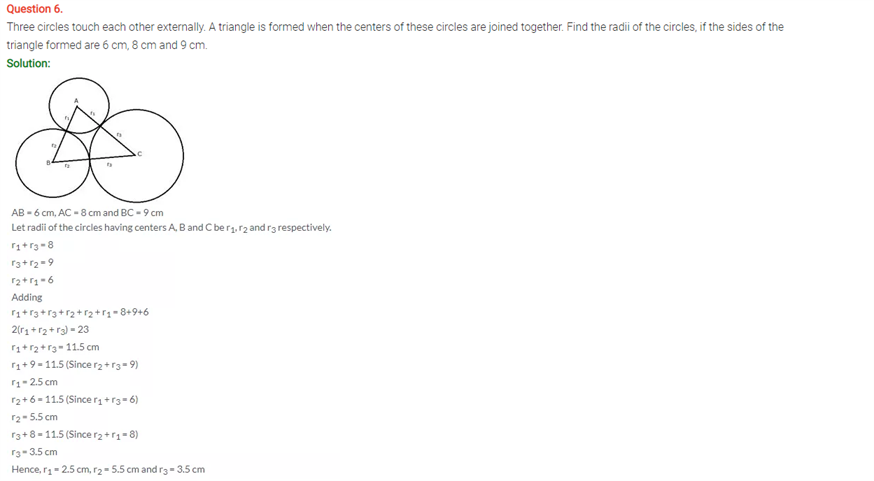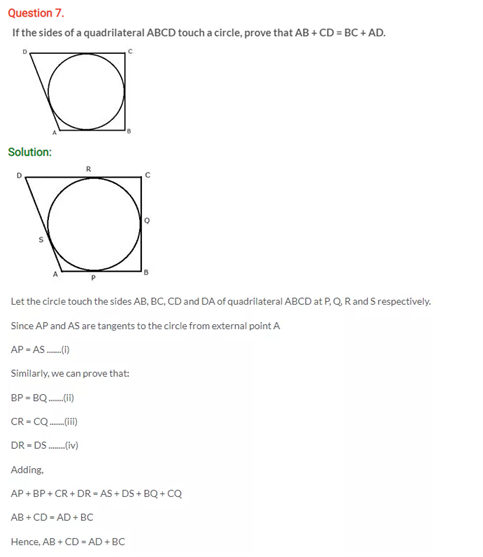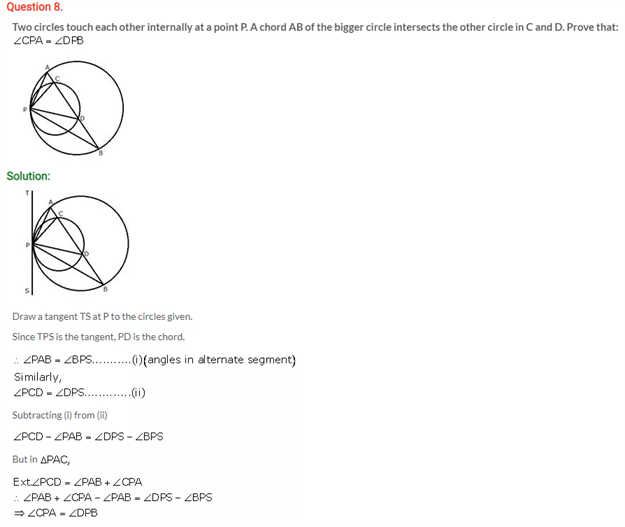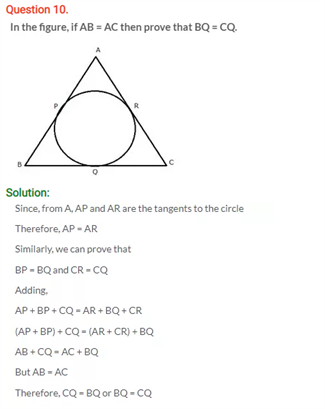# Selina Concise Maths Solution for ICSE Class 10 Chapter 18 Tangents and Intersecting Chords

## Selina Class 10 Maths Solutions for Chapter 18

In a circle, a chord is a line segment connecting two points in a circle, a tangent is a line passing a circle and touching it at only one point. Solving the solutions on tangents and chords from the ICSE Solutions For Class 10 Maths will give you clarity on this chapter. A chord is a lot like a secant, but where the secant is a line stretching to infinity in both directions, a chord is a line segment that only covers the part inside the circle. A chord that passes through the center of the circle is also a diameter of the circle. A line which touches a circle or ellipse at just one point is called a tangent and the radius to the point of tangency is always perpendicular to the tangent line. These questions below belong to the above concepts.

Check out the ICSE Class 10 Maths Selina Solutions Chapter 18 Tangents and Intersecting Chords below: